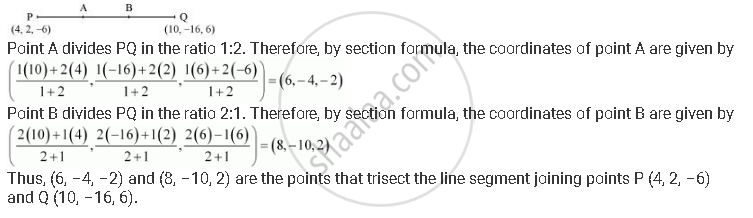# Find the Coordinates of the Points Which Trisect the Line Segment Joining the Points P (4, 2, –6) and Q (10, –16, 6). - Mathematics

Find the coordinates of the points which trisect the line segment joining the points P (4, 2, –6) and Q (10, –16, 6).

#### Solution

Let A and B be the points that trisect the line segment joining points P (4, 2, –6) and Q (10, –16, 6)Concept: Three - Dimensional Geometry - Section Formula
Is there an error in this question or solution?
Chapter 12: Introduction to Three Dimensional Geometry - Exercise 12.3 [Page 277]

#### APPEARS IN

NCERT Class 11 Mathematics
Chapter 12 Introduction to Three Dimensional Geometry
Exercise 12.3 | Q 5 | Page 277

Share